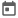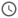# Constraints in System Verilog (Part – 1)##### 21 Oct 2023##### 6 mins

In our earlier article we learnt about the basics of randomization in System Verilog which is a powerful feature that allows us to generate random values for variables and objects. In this article, we will focus on the constraint part of randomization, which is a way to specify the conditions or restrictions on the random values. Constraint is a vast topic and thus we will discuss this topic in multiple articles.

### Need for constraints!

If we use just randomization, then any possible value for that data type can be generated. But in real life that is not ideal. There may be some valid values of that variable, or some variable should take certain set of values in some scenarios. Thus, we need to constrain our randomization such that we are generating valid scenarios.

If we are not constraining randomization then sometime, we might see unexpected behaviors from DUT side which is because input of the DUT is not valid.

For example, let’s consider an ALU with a 4-bit `op_code` input with valid values ranging from 0-9. In TB, if we randomize this 4-bit variable we can get a total of 16 possible values. But DUT accepts 0-9 as only valid values for this signal. Thus, we need to constraint our randomization so that it generates value between 0-9.

### Building blocks of constraint

Constraints can be defined in 2 ways:

• The first way is to define it inside the class where we are declaring rand variables. These are also known as named constraints as we need to provide a unique name for each constraint. These names can be used to disable certain constraints, which we will see later.

• The second way is to pass constraint while we call randomization method using with keyword, like what we have seen in earlier article. This method is also known as in-line constraints.

#### Named constraints

To write a constraint inside a class, we need to define a constraint block using `constraint` keyword and give a name to it. The body of the constraint should be enclosed within curly braces `{}`.

Unlike other blocks in SV which use `begin end` to enclose the body, constraint use `{}`. Even if we want to add a new block inside constraint like if-else, then also we need to use `{}` instead of `begin end`.

Inside the body of constraint, we provide the conditions for different random variables which will be considered while generating random variables. Each condition ends with a `;` and condition can have a logical operator like (&& or ||) as well.

##### Syntax
``````constraint <constraint_name> {
[conditions]
}``````
##### Example

For the op-code example mentioned earlier we can write constraints as follows.

``````constraint op_code_val {
op_code >= 0;
op_code <=9;
}``````

#### In-line constraint

In-line constraints are provided when we are making a call to randomize methods using `with` keyword. These are mainly used to override any of the constraints which are defined inside a class or sometimes in cases where there is no constraint provided in class but in some cases, we need to constraint the randomization.

##### Example

For op-code example, we can write in-line randomization as follows.

``    obj.randomize() with { op_code >=0 && op_code <= 9; }``

#### soft Keyword

We can use both ways to define a constraint for some random variables in single code. Now if the constraints defined inside a class and the constraints passed in-line are conflicting then SV will not be able to randomize the variable and randomization will fail.

In this scenario `soft` keyword comes in rescue. If any of the constraint, we use a condition with `soft` keyword then that condition will be given lower priority. Thus, the conflict can be resolve and SV will be able to randomize the variable.

Please note we use `soft` keyword with the condition present inside the constraint and not the constraint itself. Thus, we need to use `soft` keyword for all the conditions which are causing conflict.
##### Example

In our ALU example, let’s suppose we want to generate an error scenario where we want opcode to take invalid values.

``````constraint op_code_val {
op_code > = 0;
op_code <= 9; // randomization will fail due to this
soft op_code <= 9; // randomization will be successfull
}
...
...
obj.randomize() with { op_code > 9; }``````

#### solve-before keyword

In most of the complex scenarios we would want some random variable to take value depending on value generated for some another random variable. For this type of scenario, we would want the randomization solver to solve the constraint for the random variable on which another variable depends first.

By default, randomization can be solved in any order and thus, we need to explicitly mention if some random variable needs to be solved before another. This can be achieved using `solve-before` keyword.

##### Example

Continuing our ALU example, let’s suppose now we want to generate op_code depending upon whether it is error scenario or not. We use another random variable `error` to show that this is error scenario and thus we would want error to be solved before op_code.

``````constraint op_code_val {
solve error before op_code;
if(error) {
op_code > = 9;
}
else {
op_code >= 0;
op_code <= 9;
}
}``````
Please note that the condition in constraint is a two-way constraint. This means that if `op_code` is solved first and it takes a value of 10, then error will be 1 as `op_code` cannot greater than 9 when error is 0. Thus, we may think that the overall generated variables would be same without using solve before as well. But solve before will change the probability of generated values.

### Disabling constraints

System Verilog provides an in-build method `constraint_mode()` to enable or disable a constraint. When we pass 0 as the argument for this method, constraint will be disabled and not be used by solver.

To disable a constraint, we use `constraint_mode ()` with named constraint.

Syntax - `<constraint_name>.constraint_mode(0);`

If we do not pass any argument with the constraint_mode() method, then this method returns where the constraint is enabled or not.

#### Use-case

The following are some common usages for disabling constraints.

• This is used when we want a certain constraint to be disabled. Other constraints will still be used by SV to solve value for random variable.
• This can also be used to turn off certain constraints and pass in-line constraint which may conflict with original constraint. This enables to override constraint without using soft keywords. Though this cannot be used when we have multiple conditions in a constraint, and we want to override only one of the conditions.

### Example

``````class packet;
rand bit error;
rand bit [3:0] op_code;
rand bit [7:0] a, b;
constraint op_code_val {
solve error before op_code;
if(error) {
op_code > 9;
}
else {
op_code <= 9;
}
}
constraint a_b_val {
soft a % 2 == 0;
b % 2 == 1;
}

constraint a_grt_b {
solve b before a;
a > b;
}
function void pre_randomize();
if(a_grt_b.constraint_mode())
\$display("a_grt_b is enabled");
else
\$display("a_grt_b is disabled");
endfunction

function void printf();
\$display("Errpr = %0b, op_code = %0d, a = %0d, b = %0d", error, op_code, a, b);
endfunction
endclass //packet
module constraint_basic;
packet p;
initial begin
p = new;
p.randomize();
p.printf();

p.randomize() with {a % 2 == 1;};
p.printf();

// overriding constraint without using soft keyword
p.a_grt_b.constraint_mode(0);
p.randomize() with { b > a; };
p.printf();

// re-enabling constraint
p.a_grt_b.constraint_mode(1);
p.randomize();
p.printf();
end
endmodule``````
Try this code in EDA Playground
##### Output
``````# a_grt_b is enabled
# Errpr = 1, op_code = 13, a = 238, b = 49
# a_grt_b is enabled
# Errpr = 1, op_code = 13, a = 205, b = 151
# a_grt_b is disabled
# Errpr = 0, op_code = 2, a = 236, b = 239
# a_grt_b is enabled
# Errpr = 1, op_code = 13, a = 252, b = 201
``````

### Conclusion

In this article, we have learned how to use constraints in System Verilog to specify the conditions or restrictions on the random values generated by randomization. Constraints are a useful feature that can help us to generate valid and diverse inputs for our DUT and improve the quality of our verification.
We have learned two ways to define constraints: named constraints and in-line constraints. We have also learned some keywords to control the order, priority, and mode of the constraints, such as solve-before, soft, and constraint_mode. Additionally, we have looked at some examples of how to use constraints for different scenarios, such as ALU operation and packet generator. In the next article we will explore some advanced topics of constraint which will help us fine grain our constraints to achieve precise control on generated value.